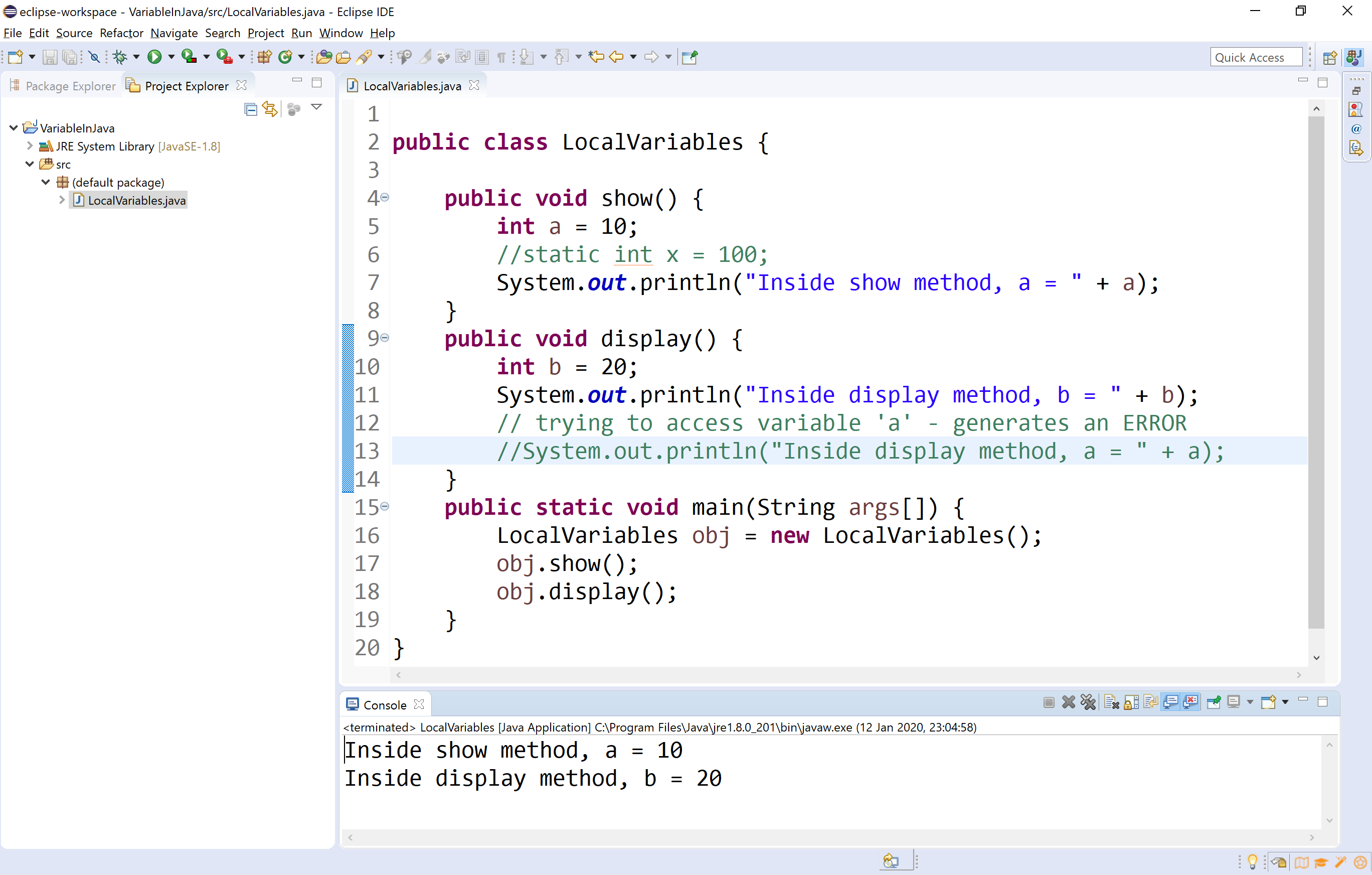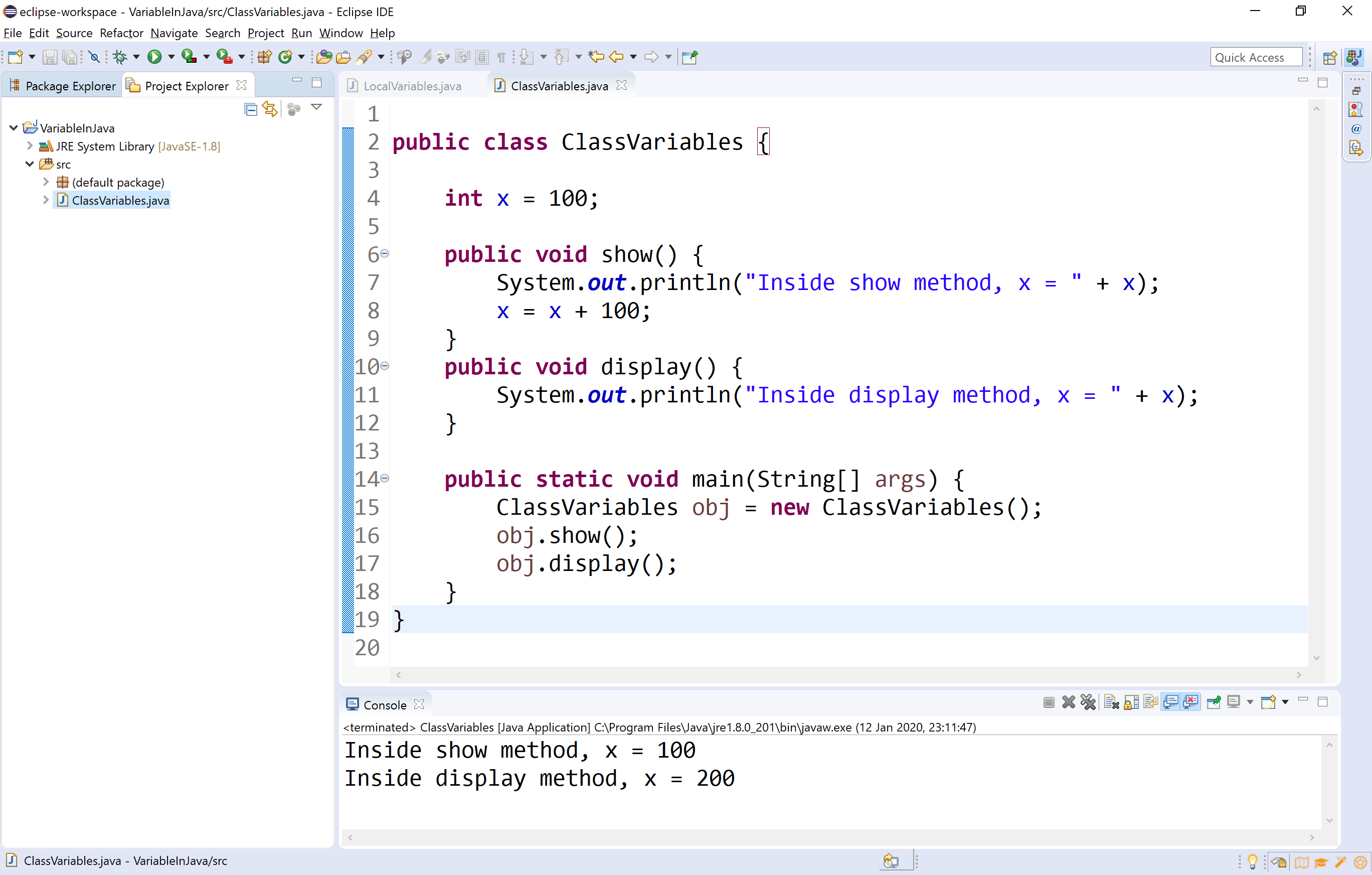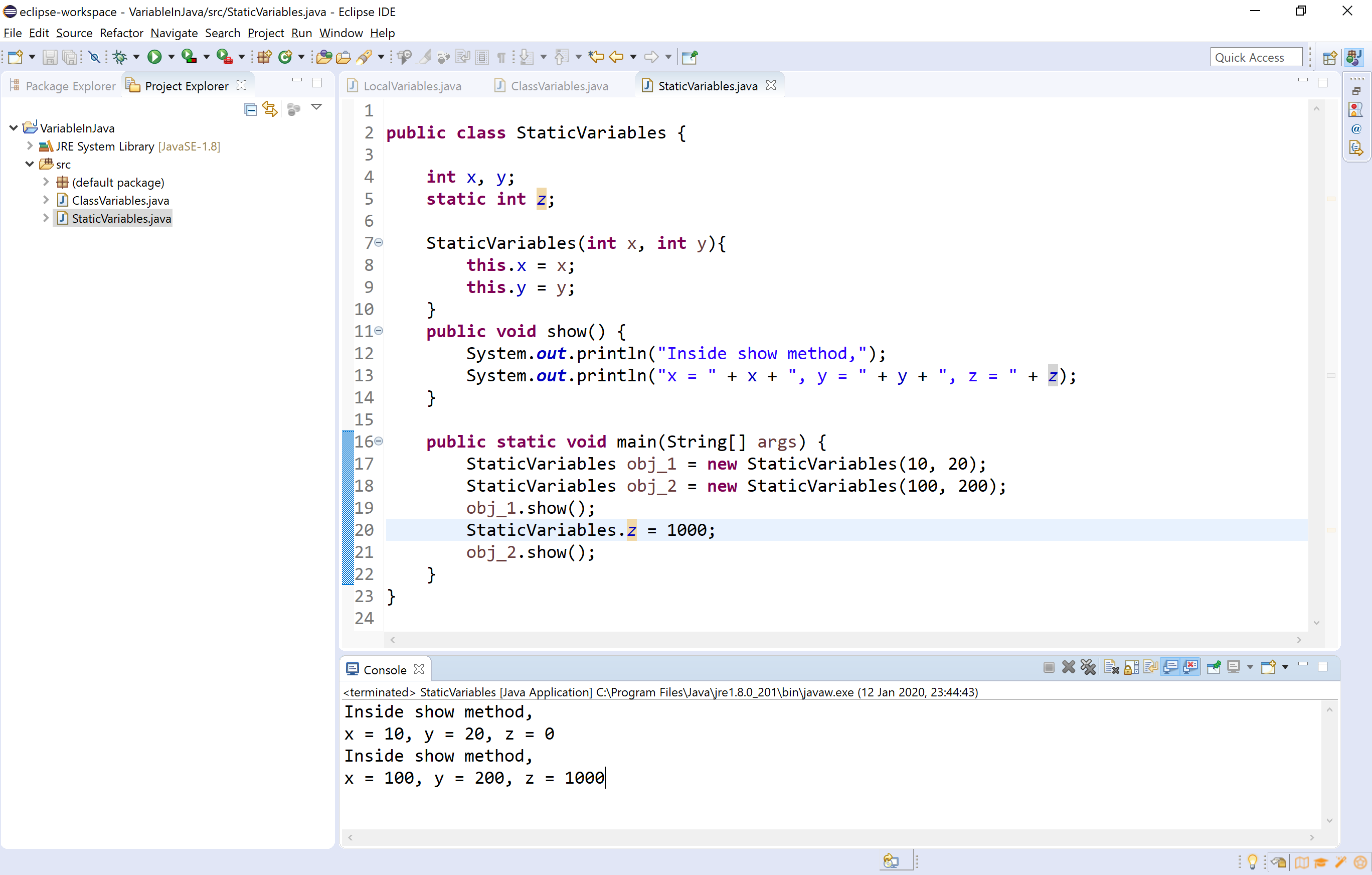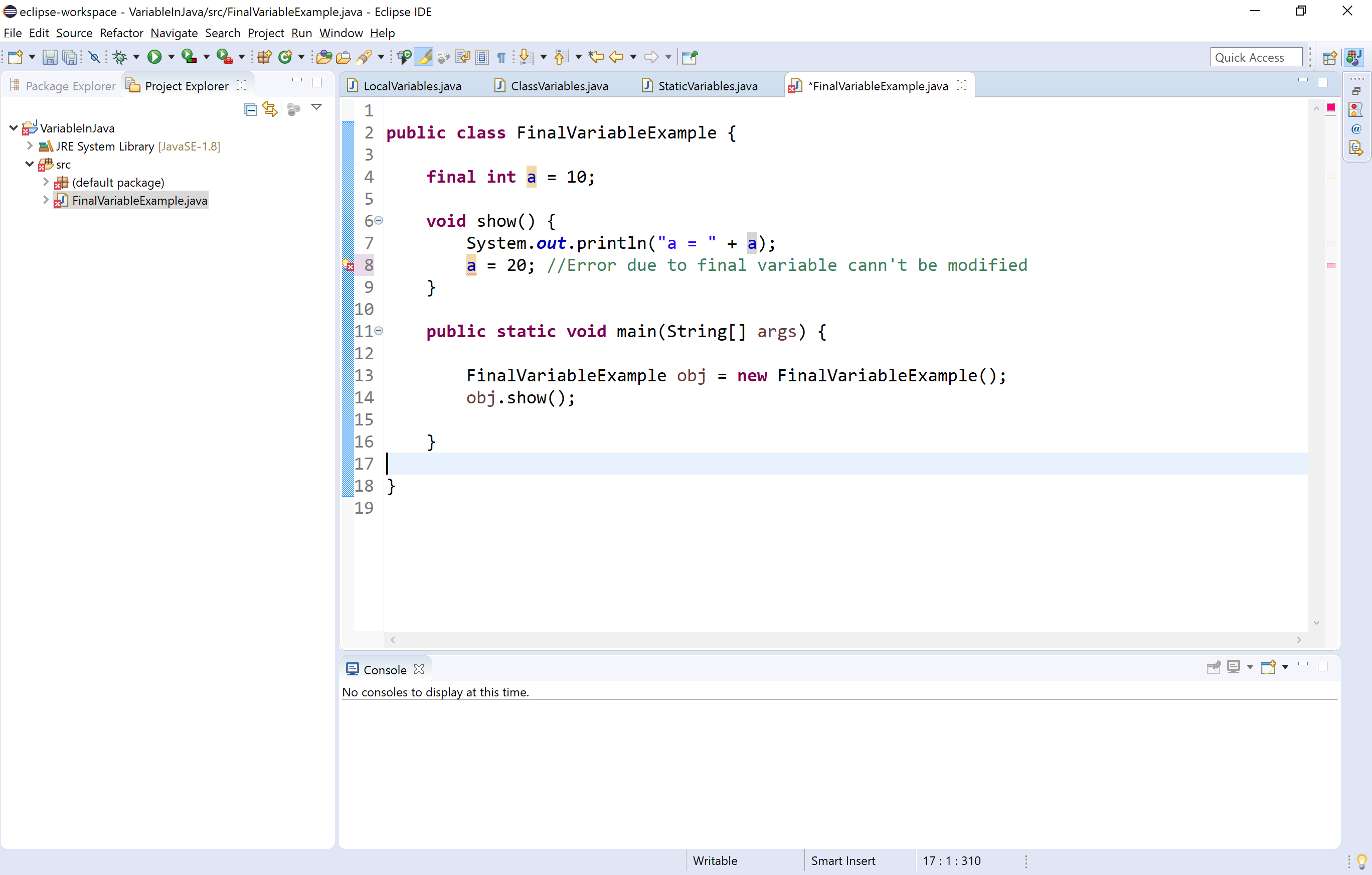The perfect place for easy learning...

×

# Java Variables

A variable is a named memory location used to store a data value. A variable can be defined as a container that holds a data value.

In java, we use the following syntax to create variables.

Syntax
``````data_type variable_name;
(or)
data_type variable_name_1, variable_name_2,...;
(or)
data_type variable_name = value;
(or)
data_type variable_name_1 = value, variable_name_2 = value,...;
``````

In java programming language variables are clasiffied as follows.

• Local variables
• Instance variables or Member variables or Global variables
• Static variables or Class variables
• Final variables

## Local variables

The variables declared inside a method or a block are known as local variables. A local variable is visible within the method in which it is declared. The local variable is created when execution control enters into the method or block and destroyed after the method or block execution completed.

Let's look at the following example java program to illustrate local variable in java.

Example
``````public class LocalVariables {

public void show() {
int a = 10;
//static int x = 100;
System.out.println("Inside show method, a = " + a);
}
public void display() {
int b = 20;
System.out.println("Inside display method, b = " + b);
// trying to access variable 'a' - generates an ERROR
System.out.println("Inside display method, a = " + a);
}
public static void main(String args[]) {
LocalVariables obj = new LocalVariables();
obj.show();
obj.display();
}
}
``````

When we run the above example code, it produces the following output.## Instance variables or member variables or global variables

The variables declared inside a class and outside any method, constructor or block are known as instance variables or member variables. These variables are visible to all the methods of the class. The changes made to these variables by method affects all the methods in the class. These variables are created separate copy for every object of that class.

Let's look at the following example java program to illustrate instance variable in java.

Example
``````public class ClassVariables {

int x = 100;

public void show() {
System.out.println("Inside show method, x = " + x);
x = x + 100;
}
public void display() {
System.out.println("Inside display method, x = " + x);
}

public static void main(String[] args) {
ClassVariables obj = new ClassVariables();
obj.show();
obj.display();
}
}
``````

When we run the above example code, it produces the following output.## Static variables or Class variables

A static variable is a variable that declared using static keyword. The instance variables can be static variables but local variables can not. Static variables are initialized only once, at the start of the program execution. The static variable only has one copy per class irrespective of how many objects we create.

The static variable is access by using class name.

Let's look at the following example java program to illustrate static variable in java.

Example
``````public class StaticVariablesExample {

int x, y;  // Instance variables
static int z;  // Static variable

StaticVariablesExample(int x, int y){
this.x = x;
this.y = y;
}
public void show() {
int a;  // Local variables
System.out.println("Inside show method,");
System.out.println("x = " + x + ", y = " + y + ", z = " + z);
}

public static void main(String[] args) {
StaticVariablesExample obj_1 = new StaticVariablesExample(10, 20);
StaticVariablesExample obj_2 = new StaticVariablesExample(100, 200);
obj_1.show();
StaticVariablesExample.z = 1000;
obj_2.show();
}
}
``````

When we run the above example code, it produces the following output.## Final variables

A final variable is a variable that declared using final keyword. The final variable is initialized only once, and does not allow any method to change it's value again. The variable created using final keyword acts as constant. All variables like local, instance, and static variables can be final variables.

Let's look at the following example java program to illustrate final variable in java.

Example
``````public class FinalVariableExample {

final int a = 10;

void show() {
System.out.println("a = " + a);
a = 20;	//Error due to final variable cann't be modified
}

public static void main(String[] args) {

FinalVariableExample obj = new FinalVariableExample();
obj.show();

}

}
``````

When we run the above example code, it produces the following output.